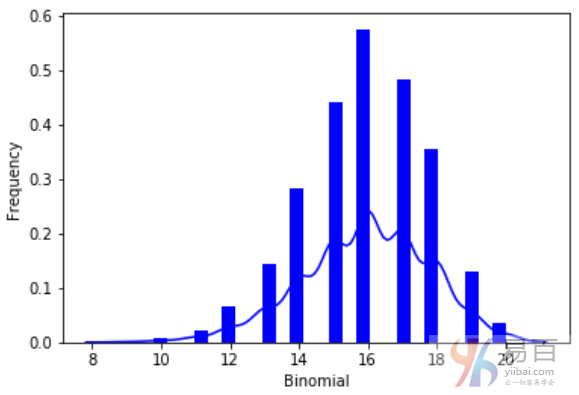# (38)Python二项分布

``````from scipy.stats import binom
import seaborn as sb

binom.rvs(size=10,n=20,p=0.8)

data_binom = binom.rvs(n=20,p=0.8,loc=0,size=1000)
ax = sb.distplot(data_binom,
kde=True,
color='blue',
hist_kws={"linewidth": 25,'alpha':1})
ax.set(xlabel='Binomial', ylabel='Frequency')
```Python```关注右侧公众号，随时随地查看教程
Python数据分析教程目录